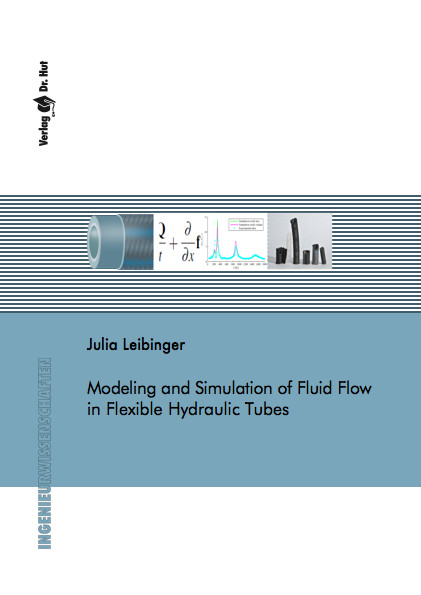Datenbestand vom 21. April 2021aktualisiert am 21. April 2021

# ISBN 978384394721360,00 € inkl. MwSt, zzgl. Versand

Julia Leibinger
Modeling and Simulation of Fluid Flow in Flexible Hydraulic Tubes

119 Seiten, Dissertation Universität Stuttgart (2020), Hardcover, A5

## Zusammenfassung / Abstract

Hydraulic systems are becoming more complex and the requirements placed on them are becoming more sophisticated. Therefore, the simulation of these systems has to become more and more precise, as it improves the understanding of the physical effects. Part of such systems are flexible tubes that connect the various other components such as throttles or pumps. Until now, simple models have been used in 1D simulation to take into account the flexible properties of the tube wall, knowing that these simple models cannot represent the behavior of all real materials.

In this thesis, therefore, models that are more complex are derived from simple elasticity models such as Hooke or Maxwell. These models contain both visco-elastic effects, as they occur for example in polymers, and direction-dependent orthotropic effects, as they occur for example in tissue structures. These effects are combined in a material model to map the behavior of complex two-layer tubes, in which the inner layer is assumed visco-elastic and the outer layer is assumed to be orthotropic. The fluid is described by the one-dimensional Euler equations, which were extended by the cross section. These equations are calculated in the present work with a finite volume method. For this purpose, the Dumbser-Osher-Toro Riemann solvers for conservative and non-conservative hyperbolic equations are combined and supplemented by a method for the treatment of stiff source terms.

To validate the method and equations, Riemann problems are solved and partly compared to a quasi-exact solution, which shows a good agreement. Finally, the results of the simulation are compared with measurement data of real tubes. It is shown that the simulation is able to reproduce the physical effects occurring in two layered tubes. Thus, the physical model and the numerical solver developed in this thesis can be used for the simulation of flexible tubes in hydraulic systems, which could not be calculated by now.